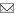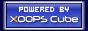Welcome Guest
www.GrammaticalPhysics.ac

Register now!
Access Detector
1 user(s) are online (1 user(s) are browsing Forum)

Members: 0
Guests: 1

more...

# Quantum Field Theory on the Time-Axis

SourceCodeOf_HumanGenome > Quantum Field Theory on the Time-Axis @ 2012/5/6 15:20
This topic is dedicated to discussion of operator formulation of the new grammar version of quantum mechanics.

It can be called quantum field theory on the time-axis.

It will be used to imitate the derivation of Feynman's path integral in the new grammar version of quantum mechanics.
---
Last edited at 2012/06/22/15:13JST
SourceCodeOf_HumanGenome > Path Integral @ 2012/5/6 15:30
|χ> is a functional such that |χ>[χ'] = δ[χ'-χ].
<χ| is a linear functional such that <χ|Φ = Φ[χ], and so <χ|χ'> = |χ'>[χ] = δ[χ-χ'].

[∫Dχ|χ><χ|Φ][χ']=∫Dχ(<χ|Φ)|χ>[χ']=∫Dχ Φ[χ]δ[χ'-χ]=Φ[χ']
∴ ∫Dχ|χ><χ|Φ=Φ
∴ ∫Dχ|χ><χ|=1
where
∫Dχ=limn→∞Πk=1n∫dχ(kT/n).

[X(t)Φ][χ] = χ(t)Φ[χ]

[X(t)|χ>][χ'] = χ'(t)|χ>[χ'] = χ'(t)δ[χ-χ'] = χ(t)δ[χ-χ'] = χ(t){|χ>[χ']} = [χ(t)|χ>][χ']
∴ X(t)|χ> = χ(t)|χ>

[P(t)Φ][χ] = -(ih/α)[δ/δχ(t)]Φ[χ]

{[X(t),P(t')]-Φ}[χ]
={[X(t)P(t')-P(t')X(t)]Φ}[χ]
={X(t)P(t')Φ-P(t')X(t)Φ}[χ]
=[X(t)P(t')Φ][χ]-[P(t')X(t)Φ][χ]
={X(t)[P(t')Φ]}[χ]-{P(t')[X(t)Φ]}[χ]
=χ(t)[P(t')Φ][χ]-(-ih/α)[δ/δχ(t')][X(t)Φ]}[χ]
=χ(t)(-ih/α)[δ/δχ(t')]Φ[χ]-(-ih/α)[δ/δχ(t')]{χ(t)Φ[χ]}
=[χ(t),-(ih/α)[δ/δχ(t')]]-Φ[χ]
=(ih/α)δ(t-t')Φ[χ]
=[(ih/α)δ(t-t')Φ][χ]
∴ [X(t),P(t')]- = (ih/α)δ(t-t')

|π> is defined as a functional such that |π>[χ] = exp[(iα/h)∫dt π(t)χ(t)].

<χ|π>=|π>[χ]=exp[(iα/h)∫dt π(t)χ(t)]

[P(t)|π>][χ] = -(ih/α)[δ/δχ(t)]|π>[χ]
= -(ih/α)[δ/δχ(t)]exp[(iα/h)∫dt' π(t')χ(t')]
= π(t)exp[(iα/h)∫dt' π(t')χ(t')]
= π(t)|π>[χ]
= [π(t)|π>][χ]
∴ P(t)|π> = π(t)|π>

<π| is defined as a linear functional such that <π|Φ = ∫Dχ exp[-(iα/h)∫dt π(t)χ(t)]Φ[χ].

<π|χ>=∫Dχ' exp[-(iα/h)∫dt π(t)χ'(t)]|χ>[χ']
=∫Dχ' exp[-(iα/h)∫dt π(t)χ'(t)]δ[χ'-χ]
=exp[-(iα/h)∫dt π(t)χ(t)]

[∫Dπ|π><π|Φ][χ]
=∫Dπ(<π|Φ)|π>[χ]
=∫Dπ{∫Dχ' exp[-(iα/h)∫dt π(t)χ'(t)]Φ[χ']}exp[(iα/h)∫dt' π(t')χ(t')]
=∫Dχ'∫Dπ exp{(iα/h)∫dt π(t)[χ(t)-χ'(t)]}Φ[χ']
=∫Dχ' limn→∞[(α/h)(T/n)/(2×3.14・・・)]nk=1n∫dπ(kT/n)]exp{(iα/h)(T/n)Σj=1n π(jT/n)[χ(jT/n)-χ'(jT/n)]}Φ[χ']
=∫Dχ' Φ[χ']limn→∞[(α/h)(T/n)/(2π)]nΠk=1n(2π)[(α/h)(T/n)]-1δ(χ(jT/n)-χ'(jT/n))
=∫Dχ' Φ[χ']limn→∞Πk=1nδ(χ(jT/n)-χ'(jT/n))
=∫Dχ' Φ[χ']δ[χ-χ']
=Φ[χ]
∴ ∫Dπ|π><π|Φ = Φ
∴ ∫Dπ|π><π| = 1
where
∫Dπ=limn→∞[(α/h)(T/n)/(2π)]nΠk=1n∫dπ(kT/n).

<π|P(t)Φ=∫Dχ exp[-(iα/h)∫dt' π(t')χ(t')][P(t)Φ][χ]
=∫Dχ exp[-(iα/h)∫dt' π(t')χ(t')](-ih/α)[δ/δχ(t)]Φ[χ]
=∫Dχ Φ[χ](ih/α)[δ/δχ(t)]exp[-(iα/h)∫dt' π(t')χ(t')]
=∫Dχ Φ[χ]π(t)exp[-(iα/h)∫dt' π(t')χ(t')]
=π(t)<π|Φ
∴ <π|P(t) = π(t)<π|

The new grammar version of Schrödinger equation is as follows.
(ih/α)(d/dε)<χ(□-ε)|Φ>|ε=0 = <χ|∫dt{[P(t)]2/(2m)+V(X(t))}|Φ>

Φε[χ] ≡ Φ[χ(□-ε)]
<χ|Φε>=<χ(□-ε)|Φ>
(ih/α)(d/dε)|Φε> = ∫dt{[P(t)]2/(2m)+V(X(t))}|Φε>

H=∫dt{[P(t)]2/(2m)+V(X(t))}
ε>=exp[(-iα/h)εH]|Φ0>
=limn→∞{exp[(-iα/h)(ε/n)H]}n0>
=limn→∞∫Dχ1・・・∫Dχn exp[(-iα/h)(ε/n)H]|χn><χn|・・・|χ2><χ2|exp[(-iα/h)(ε/n)H]|χ1><χ10>

k+1|exp[(-iα/h)(ε/n)H]|χk>
=∫Dπ<χk+1|π><π|exp[(-iα/h)(ε/n)H]|χk>
=∫Dπ exp[(-iα/h)(ε/n)∫dt{[π(t)]2/(2m)+V(χk(t))}]k+1|π><π|χk>
=∫Dπ exp[(-iα/h)(ε/n)∫dt{[π(t)]2/(2m)+V(χk(t))}]exp{(iα/h)∫dt π(t)[χk+1(t)-χk(t)]}
=∫Dπ exp{(iα/h)(ε/n)∫dt[π(t)[χk+1(t)-χk(t)]/(ε/n)-{[π(t)]2/(2m)+V(χk(t))}]}
=limn'→∞[(α/h)(T/n')/(2×3.14・・・)]n'Πj=1n'∫dπ(jT/n')exp{(iα/h)(ε/n)(T/n')Σs=1n'[-[1/(2m)]{π(sT/n')-m[χk+1(sT/n')-χk(sT/n')]/(ε/n)}2-V(χk(sT/n'))+(m/2){[χk+1(sT/n')-χk(sT/n')]/(ε/n)}2]}
=limn'→∞[(α/h)(T/n')/(2×3.14・・・)]n'{(2m×3.14・・・)/[(iα/h)(ε/n)(T/n')]}n'/2exp[(iα/h)(ε/n)∫dt{(m/2){[χk+1(t)-χk(t)]/(ε/n)}2-V(χk(t))}]
=limn'→∞{(α/h)(T/n')m/[2i(ε/n)×3.14・・・]}n'/2exp[(iα/h)(ε/n)∫dt{(m/2){[χk+1(t)-χk(t)]/(ε/n)}2-V(χk(t))}]
=limn'→∞{(α/h)(T/n')m/[2πi(ε/n)]}n'/2exp[(iα/h)(ε/n)∫dt{(m/2){[χk+1(t)-χk(t)]/(ε/n)}2-V(χk(t))}]

C≡limn'→∞{(α/h)(T/n')m/[2πi(ε/n)]}n'/2=0
This is a difficulty!

ξ(kε/n, t)≡χk(t)

k+1|exp[(-iα/h)(ε/n)H]|χk>
=C exp[(iα/h)(ε/n)∫dt{(m/2)[(d/dτ)ξ(τ,t)]2-V(ξ(τ,t))}]|τ=kε/n (n→∞)

n+1ε>=∫Dξexp[(iα/h)∫0εdτ∫0Tdt{(m/2)[(d/dτ)ξ(τ,t)]2-V(ξ(τ,t))}]<ξ(ε/n,□)|Φ0>
where
∫Dξ=limn→∞Cnlimn'→∞Πk=1nΠj=1n'∫dξ(kε/n,jT/n')
=limn→∞limn'→∞{(α/h)(T/n')m/[2πi(ε/n)]}nn'/2Πk=1nΠj=1n'∫dξ(kε/n,jT/n')
Here the difficulty is absorbed into the functional integral measure.

Φε[ξ(ε,□)] = ∫Dξ exp[(iα/h)∫0εdτ∫0Tdt{(m/2)[(d/dτ)ξ(τ,t)]2-V(ξ(τ,t))}]Φ0[ξ(0,□)]

Φε[ξ(ε,□)] = Φ[ξ(ε,□-ε)]

Φ[ξ(ε,□-ε)] = ∫Dξ exp[(iα/h)∫0εdτ∫0Tdt{(m/2)[(d/dτ)ξ(τ,t)]2-V(ξ(τ,t))}]Φ[ξ(0,□)]

This message follows the idea on 2012/05/01.
---
Last edited at 2012/07/18/14:53JST
SourceCodeOf_HumanGenome > Interpretation of the Path Integral @ 2012/6/22 15:32
Though it is impossible because entanglement is inevitable, if
Φ[χ] = limn→∞ Πk=1n φ(χ(kT/n),kT/n),
the conclusion of the previous message is understood as follows.

φ(ξ(ε,kT/n),kT/n+ε)
= ∫Dξ(□,kT/n) exp[(iα/h)(T/n)∫0ε{(m/2)[(d/dτ)ξ(τ,kT/n)]2-V(ξ(τ,kT/n))}]φ(ξ(0,kT/n),kT/n)

Φ[ξ(ε,□-ε)] = limn→∞ Πk=1n φ(ξ(ε,kT/n-ε),kT/n) = limn→∞ Πk=1n φ(ξ(ε,kT/n),kT/n+ε),
Φ[ξ(0,□)] = limn→∞ Πk=1n φ(ξ(0,kT/n),kT/n).

Therefore,
φ(χ(ε),kT/n+ε) = ∫Dχ exp[(iα/h)(T/n)∫0ε{(m/2)[(d/dτ)χ(τ)]2-V(χ(τ)}]φ(χ(0),kT/n).

This means that φ obeys the ordinary Schrodinger equation when T/n=1/α, and so the new theory is natural extension of the old one.
That is to say, the length of an instant which defines a quantum state is 1/α.

Then we can write an approximate solution as follows.
G(χ(ε),ε;χ(0),0) = ∫Dχ exp[(i/h)∫0ε{(m/2)[(d/dτ)χ(τ)]2-V(χ(τ)}],
φ(χ(ε),k/α+ε) = ∫dχ(0) G(χ(ε),ε;χ(0),0) φ(χ(0),k/α)　(k=1,2,・・・,αT),
Φ[χ] = Πk=1αT φ(χ(k/α),k/α)
where G is the Green's function.
---
Last edited at 2012/07/17/15:43JST
SourceCodeOf_HumanGenome > Probability Interpretation @ 2012/6/22 16:25
When
Φ[χ] = ∫dx Πk=1αT G(χ(k/α),k/α;x,t0),
χ(a)=A,χ(b)=BDχ Φ[χ] ≠ C G(A,a;B,b)

---
Last edited at 2012/07/21/14:42JST
SourceCodeOf_HumanGenome > A Sketch of an Entangled Solution @ 2012/7/3 14:42
Consider the case that T/n = 1/α and the functional:
Φ[χ] = ∫dx Πk=1αT G(χ(k/α),k/α;x,t0)
where G is the Green's function.

This functional Φ is a sum of quantum histories which give x for measurement at time t0.

∫Dξ exp[(iα/h)∫0εdτ∫0Tdt{(m/2)[(d/dτ)ξ(τ,t)]2-V(ξ(τ,t))}]Φ[ξ(0,□)]
≒ ∫Dξ exp[(iα/h)(T/n)Σk=1n0εdτ{(m/2)[(d/dτ)ξ(τ,kT/n)]2-V(ξ(τ,kT/n))}]Φ[ξ(0,□)]
= ∫Dξ Πk=1αTexp[(i/h)∫0εdτ{(m/2)[(d/dτ)ξ(τ,k/α)]2-V(ξ(τ,k/α))}]Φ[ξ(0,□)]
= [Πk=1αT∫dξ(0,k/α) G(ξ(ε,k/α),ε;ξ(0,k/α),0)]Φ[ξ(0,□)]
= [Πk=1αT∫dξ(0,k/α) G(ξ(ε,k/α),ε;ξ(0,k/α),0)]∫dx Πk=1αT G(ξ(0,k/α),k/α;x,t0)
= ∫dx Πk=1αT∫dξ(0,k/α) G(ξ(ε,k/α),ε;ξ(0,k/α),0) G(ξ(0,k/α),k/α;x,t0)
= ∫dx Πk=1αT G(ξ(ε,k/α),k/α+ε;x,t0)
= ∫dx Πk=1αT G(ξ(ε,k/α-ε),k/α;x,t0)
= Φ[ξ(ε,□-ε)]

Therefore,
Φ[χ] = ∫dx Πk=1αT G(χ(k/α),k/α;x,t0)
is an approximate solution.

∫dx is not necessary, but I expect that the entanglement caused by it rationalizes the probability interpretation.

This message follows the idea on 2012/07/02.
---
Last edited at 2012/07/22/11:04JST
Site Search
Site Info
Webmasterswooder